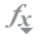## Working with Member Formulas

You can define or edit member formulas directly in the Simplified dimension editor grid, in the formula bar, or in the Member Formula dialog where you can validate member formulas.

You can define member formulas to combine operators, calculation functions, dimension and member names, and numeric constants to perform calculations on members. Member formulas can also include:

• Operator type, function, value, member name, UDA, and so on allowed in formulas.

• Predefined formula expressions, including Smart List values, that expand into a formula or value upon database refresh.

Best Practices:

• Use member formulas for Level 0 members only.

• Avoid using member formulas on Custom dimensions, unless required for reporting.

• Avoid using member formulas on the Movement dimension, due to consolidation performance considerations.

• Two-pass calculations are recommended to be used on the Account dimension only.

• Custom member formulas should not be added under the Total Balance Sheet hierarchy.

• Level 0 members should not be Dynamic Calc without member formulas.

To define or edit member formulas in the Simplified dimension editor:

1. View Edit Member Properties.
2. In the Default Formula column of the grid, select the member for which you want to define or edit a formula. Define or edit the formula for the member using one of the following options:
• Click the cell once more in the dimension editor grid to enter or edit the formula.

• Click within the formula bar above the dimension editor grid, and then enter or edit the formula.

• Clicknext to the formula bar, and then enter or edit the formula.

Tip:

To include member names in formulas, keep the focus on the formula cell in the grid. Press Ctrl while clicking the member name you want to include in the formula. The member name will display in the formula bar.

3. Optional: To check the validity of a member formula, clicknext to the formula bar, and then click Validate.
4. Click Save.